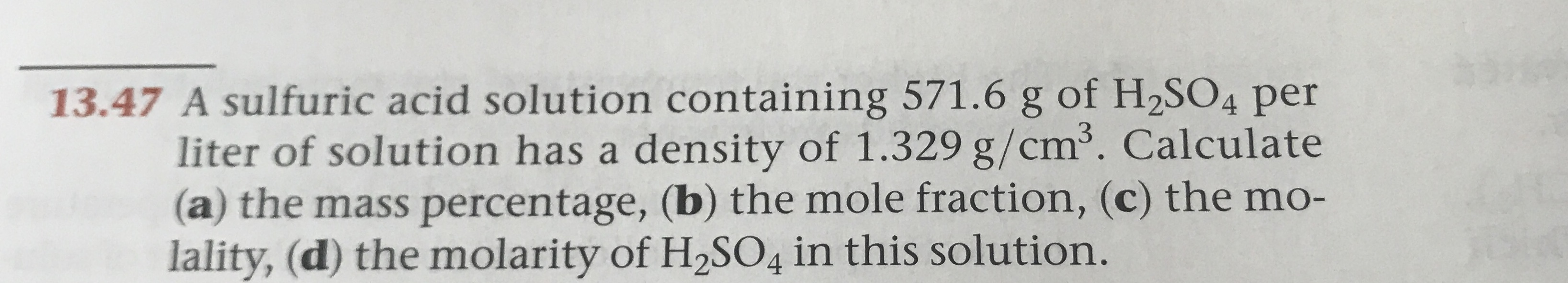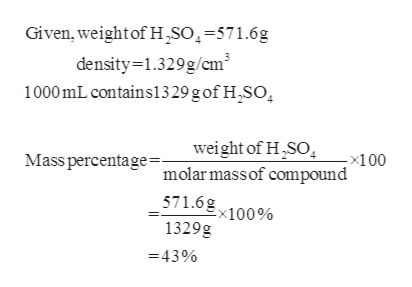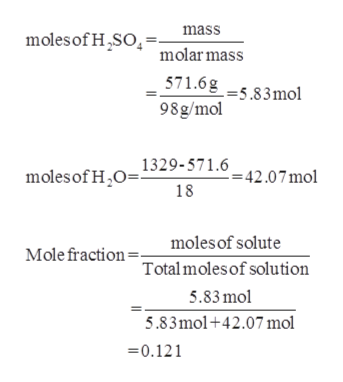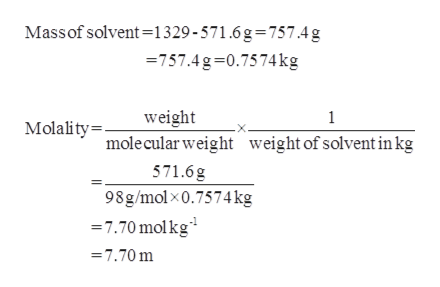# 13.47 A sulfuric acid solution containing 571.6 g of H2SO4 perliter of solution has a density of 1.329 g/cm2. Calculate(a) the mass percentage, (b) the mole fraction, (c) the mo-lality, (d) the molarity of H2SO4 in this solution.

Question
1 viewshelp_outlineImage Transcriptionclose13.47 A sulfuric acid solution containing 571.6 g of H2SO4 per liter of solution has a density of 1.329 g/cm2. Calculate (a) the mass percentage, (b) the mole fraction, (c) the mo- lality, (d) the molarity of H2SO4 in this solution. fullscreen
check_circle

Step 1

(a)The mass percentage of sulfuric acid solution can be calculated ashelp_outlineImage TranscriptioncloseGiven, weight of H,SO=571.6g density-1.329g/cm3 1000mL contains1329 g of H,SO weight of H SO -x100 Mass percentage=. molarmassof compound 571.6gx100% 1329g 43% fullscreen
Step 2

(b) The mole fraction of sulfuric acid solution can be calculated ashelp_outlineImage Transcriptionclosemass moles of HSO molar mass 571.6g 98g/mol --5.83 mol 1329-571.6 moles of H20 =42.07 mol 18 moles of solute Mole fraction Totalmoles of solution 5.83mol 5.83mol+42.07 mol 0.121 fullscreen
Step 3

(c) The molality of sulfuric acid s...help_outlineImage TranscriptioncloseMassof solvent-1329-571.6g=757.4g 757.4g-0.7574kg weight 1 Molality mole cular weight weight of solvent inkg 571.6g 98g/molx0.7574 kg 7.70 molkg =7.70 m fullscreen

### Want to see the full answer?

See Solution

#### Want to see this answer and more?

Solutions are written by subject experts who are available 24/7. Questions are typically answered within 1 hour.*

See Solution
*Response times may vary by subject and question.
Tagged in

### Physical Chemistry